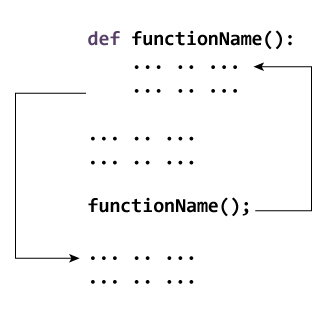# Python Functions

In this article, you'll learn about functions, what a function is, the syntax, components, and types of functions. Also, you'll learn to create a function in Python.

## What is a function in Python?

In Python, a function is a group of related statements that performs a specific task.

Functions help break our program into smaller and modular chunks. As our program grows larger and larger, functions make it more organized and manageable.

Furthermore, it avoids repetition and makes the code reusable.

### Syntax of Function

```def function_name(parameters):
"""docstring"""
statement(s)```

Above shown is a function definition that consists of the following components.

1. Keyword `def` that marks the start of the function header.
2. A function name to uniquely identify the function. Function naming follows the same rules of writing identifiers in Python.
3. Parameters (arguments) through which we pass values to a function. They are optional.
4. A colon (:) to mark the end of the function header.
5. Optional documentation string (docstring) to describe what the function does.
6. One or more valid python statements that make up the function body. Statements must have the same indentation level (usually 4 spaces).
7. An optional `return` statement to return a value from the function.

### Example of a function

``````def greet(name):
"""
This function greets to
the person passed in as
a parameter
"""
print("Hello, " + name + ". Good morning!")``````

### How to call a function in python?

Once we have defined a function, we can call it from another function, program or even the Python prompt. To call a function we simply type the function name with appropriate parameters.

``````>>> greet('Paul')
Hello, Paul. Good morning!``````

Note: Try running the above code in the Python program with the function definition to see the output.

``````def greet(name):
"""
This function greets to
the person passed in as
a parameter
"""
print("Hello, " + name + ". Good morning!")

greet('Paul')``````

## Docstrings

The first string after the function header is called the docstring and is short for documentation string. It is briefly used to explain what a function does.

Although optional, documentation is a good programming practice. Unless you can remember what you had for dinner last week, always document your code.

In the above example, we have a docstring immediately below the function header. We generally use triple quotes so that docstring can extend up to multiple lines. This string is available to us as the `__doc__` attribute of the function.

For example:

Try running the following into the Python shell to see the output.

``````>>> print(greet.__doc__)

This function greets to
the person passed in as
a parameter``````

To learn more about docstrings in Python, visit Python Docstrings.

## The return statement

The `return` statement is used to exit a function and go back to the place from where it was called.

### Syntax of return

`return [expression_list]`

This statement can contain an expression that gets evaluated and the value is returned. If there is no expression in the statement or the `return` statement itself is not present inside a function, then the function will return the `None` object.

For example:

``````>>> print(greet("May"))
Hello, May. Good morning!
None``````

Here, `None` is the returned value since `greet()` directly prints the name and no `return` statement is used.

### Example of return

``````def absolute_value(num):
"""This function returns the absolute
value of the entered number"""

if num >= 0:
return num
else:
return -num

print(absolute_value(2))

print(absolute_value(-4))``````

Output

```2
4```

## How Function works in Python?Working of functions in Python

## Scope and Lifetime of variables

Scope of a variable is the portion of a program where the variable is recognized. Parameters and variables defined inside a function are not visible from outside the function. Hence, they have a local scope.

The lifetime of a variable is the period throughout which the variable exits in the memory. The lifetime of variables inside a function is as long as the function executes.

They are destroyed once we return from the function. Hence, a function does not remember the value of a variable from its previous calls.

Here is an example to illustrate the scope of a variable inside a function.

``````def my_func():
x = 10
print("Value inside function:",x)

x = 20
my_func()
print("Value outside function:",x)``````

Output

```Value inside function: 10
Value outside function: 20```

Here, we can see that the value of x is 20 initially. Even though the function `my_func()` changed the value of x to 10, it did not affect the value outside the function.

This is because the variable x inside the function is different (local to the function) from the one outside. Although they have the same names, they are two different variables with different scopes.

On the other hand, variables outside of the function are visible from inside. They have a global scope.

We can read these values from inside the function but cannot change (write) them. In order to modify the value of variables outside the function, they must be declared as global variables using the keyword `global`.

## Types of Functions

Basically, we can divide functions into the following two types:

1. Built-in functions - Functions that are built into Python.
2. User-defined functions - Functions defined by the users themselves.